Examples

Chapter 10 Class 11 Straight Lines
Serial order wise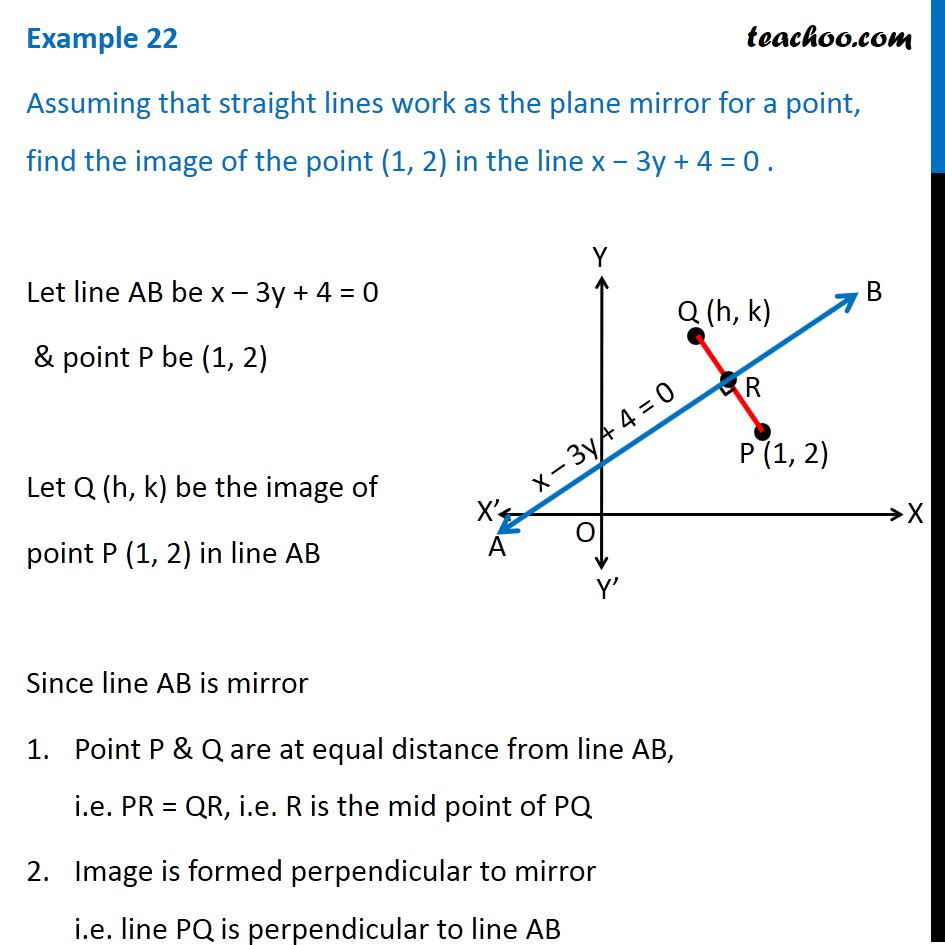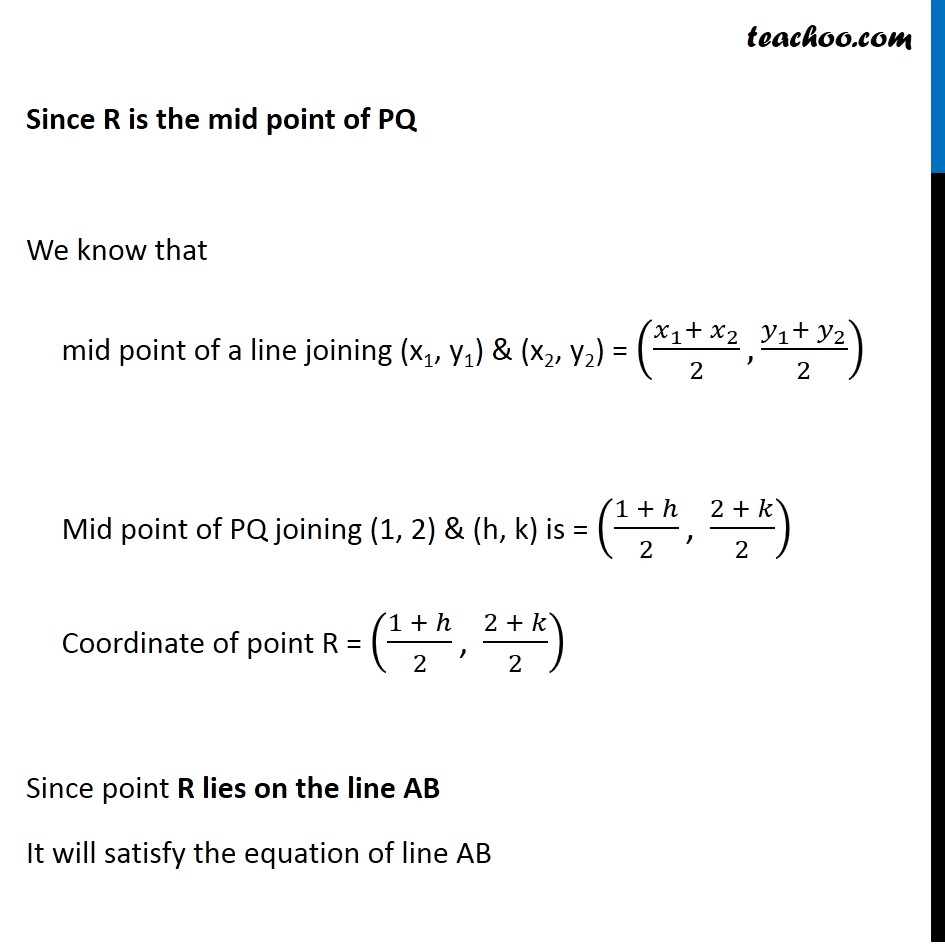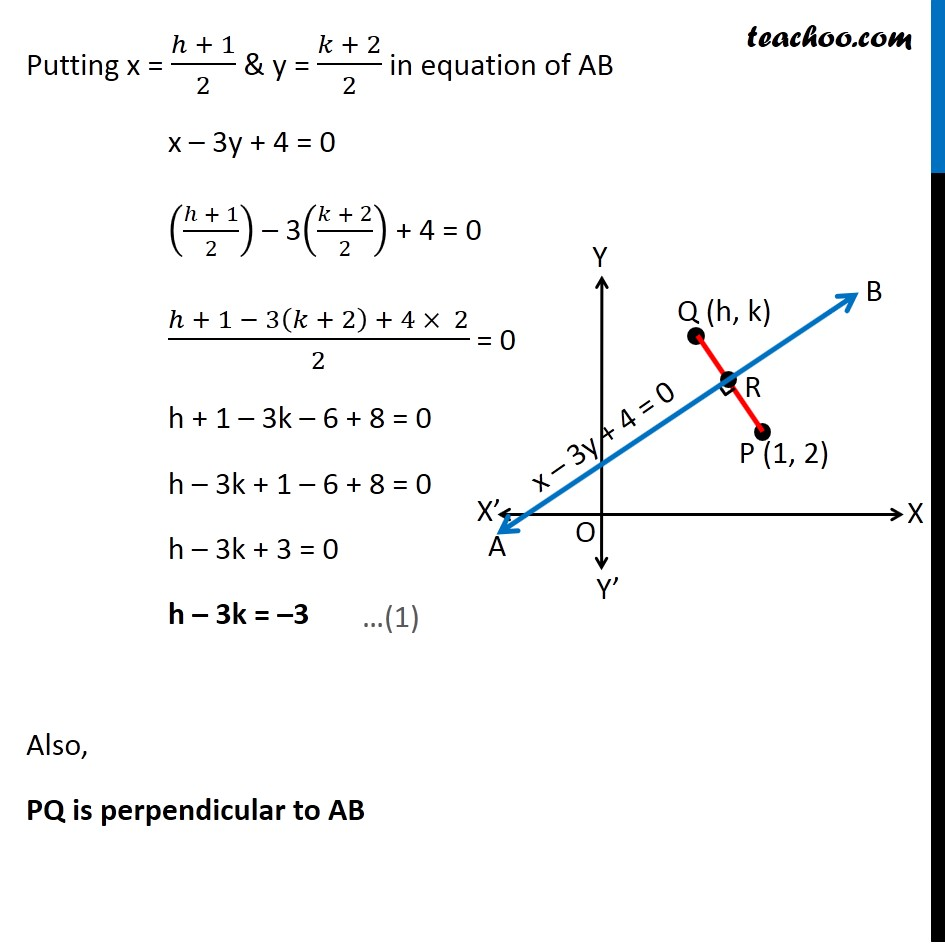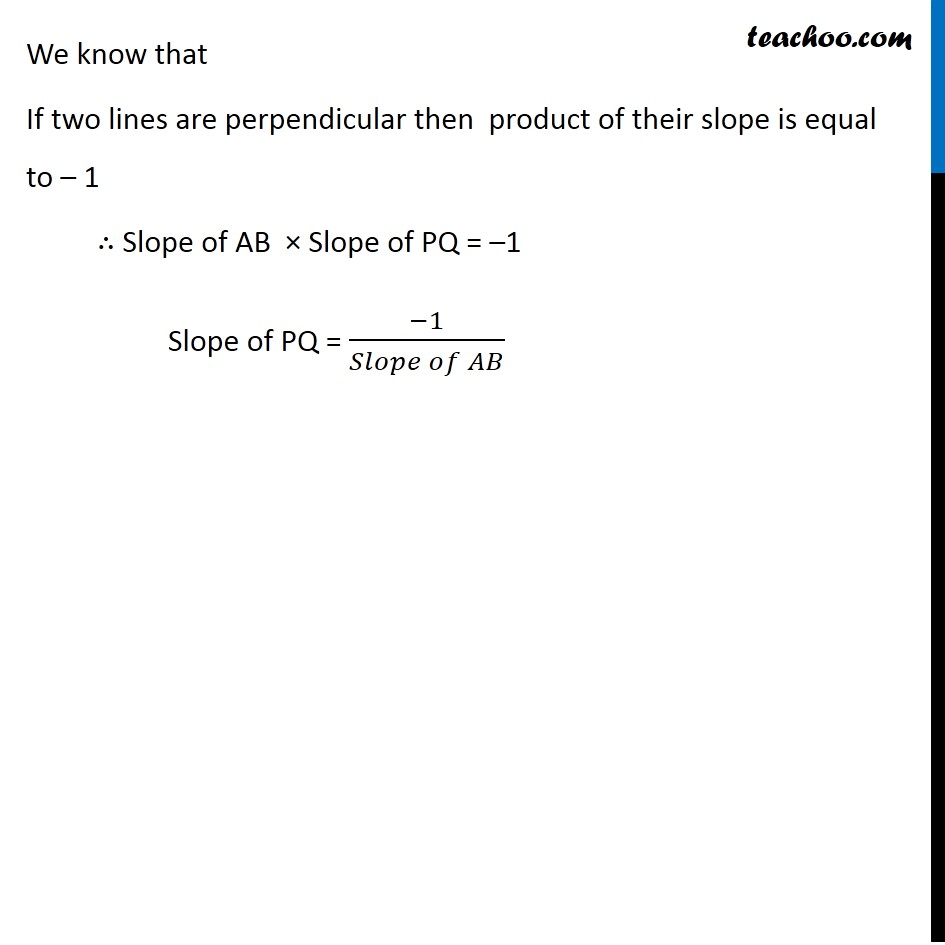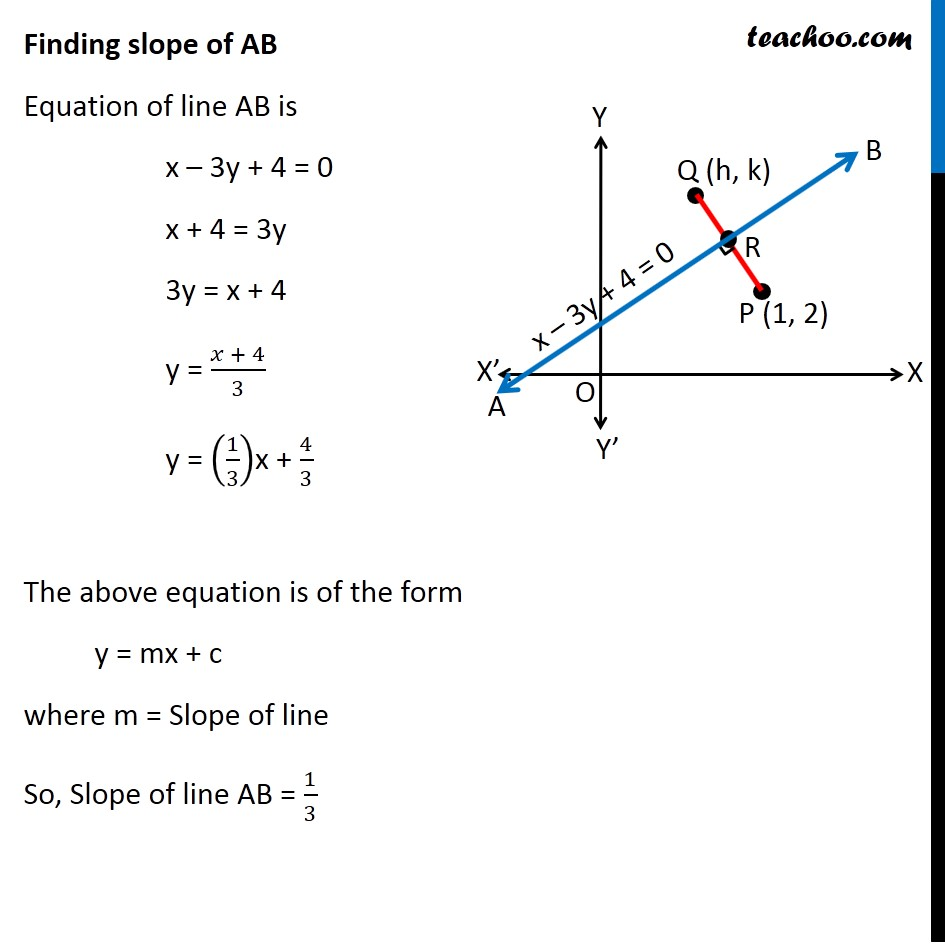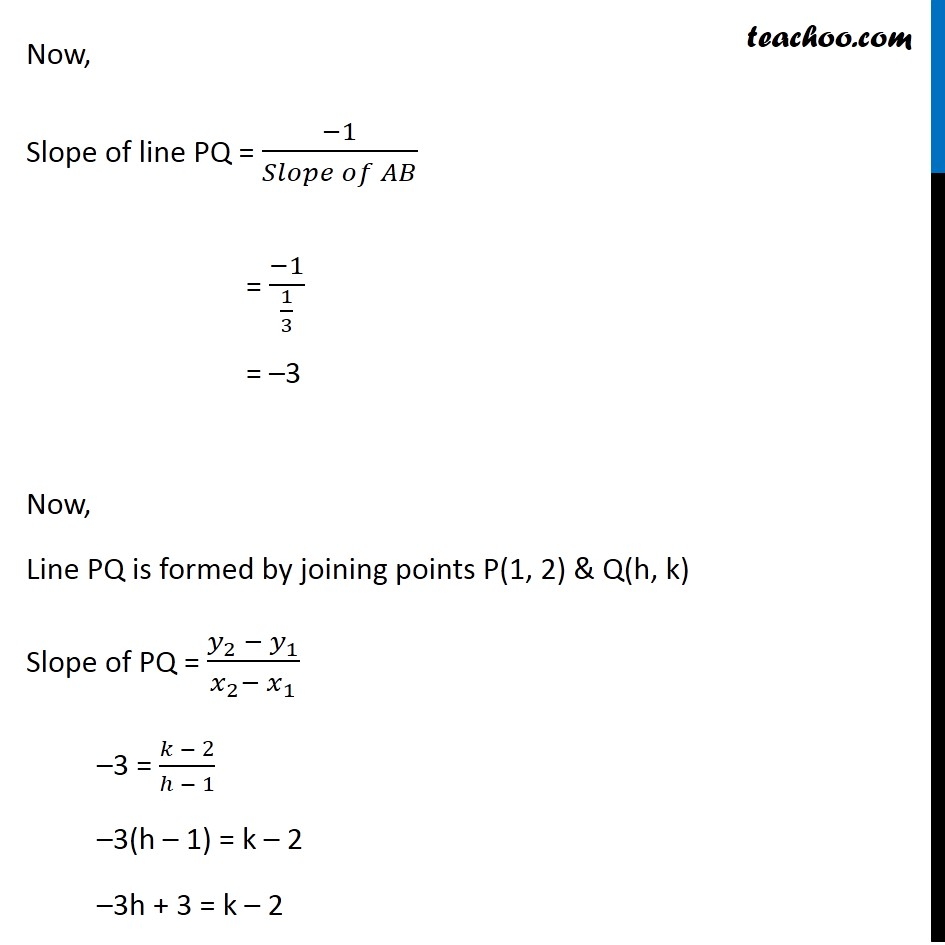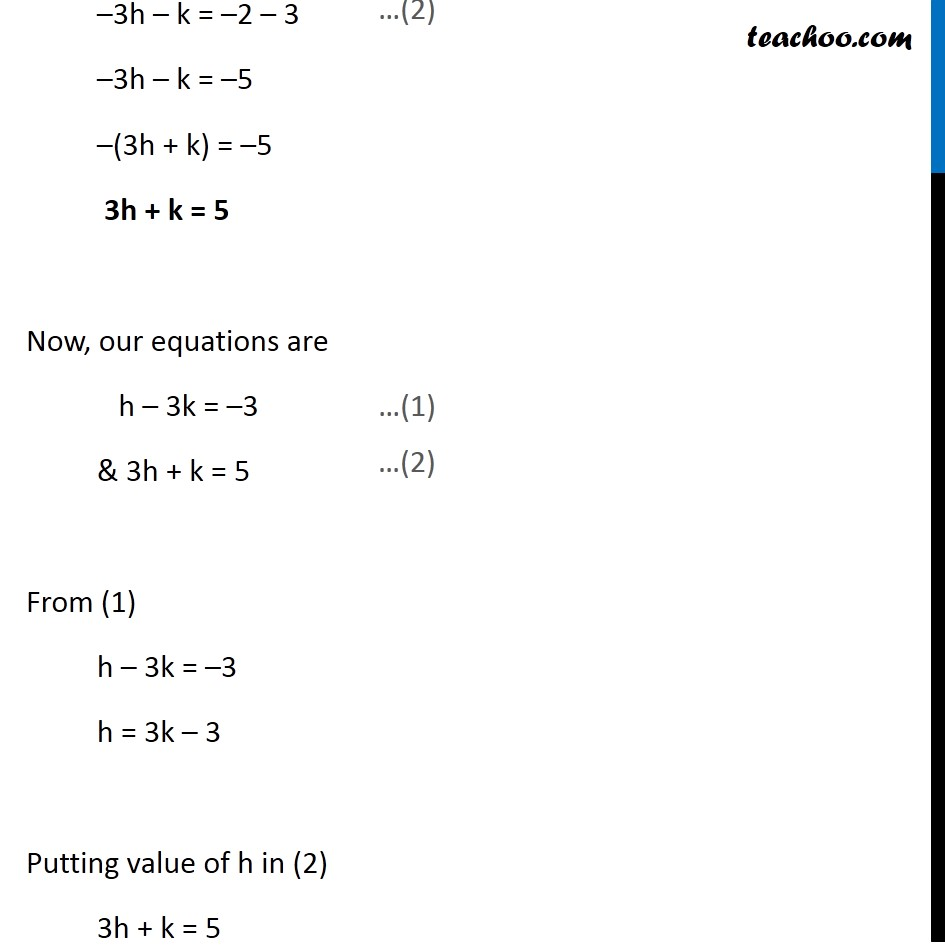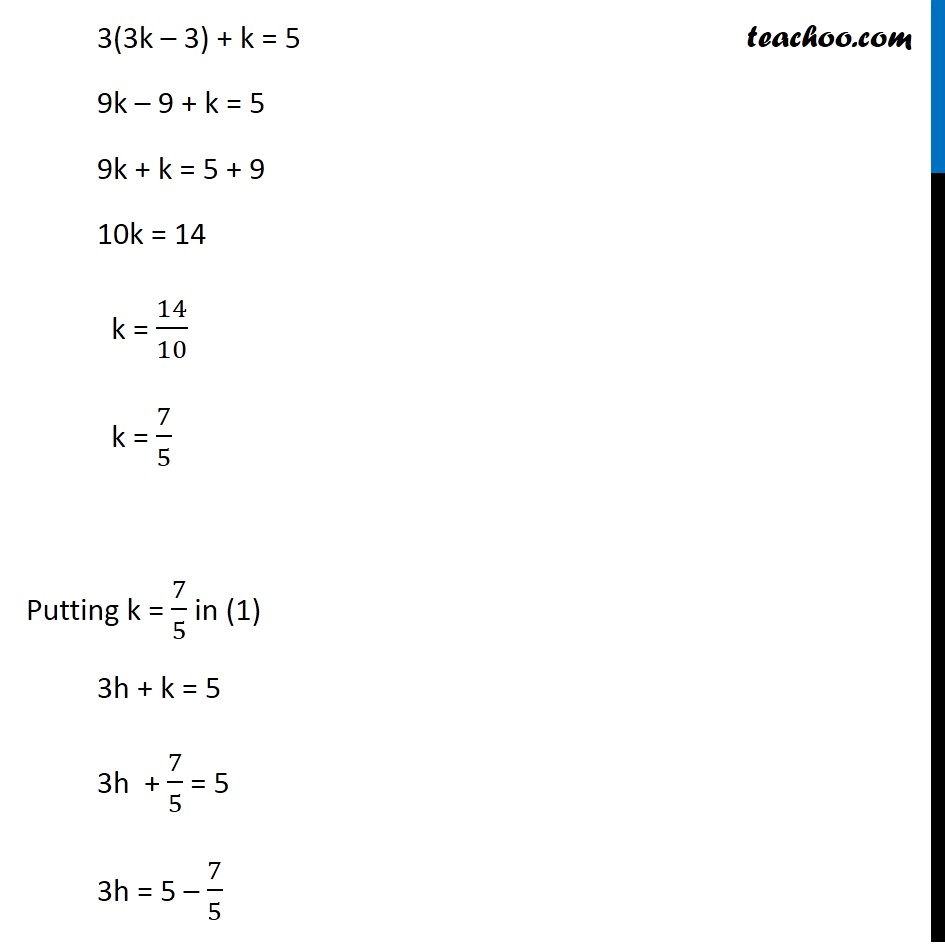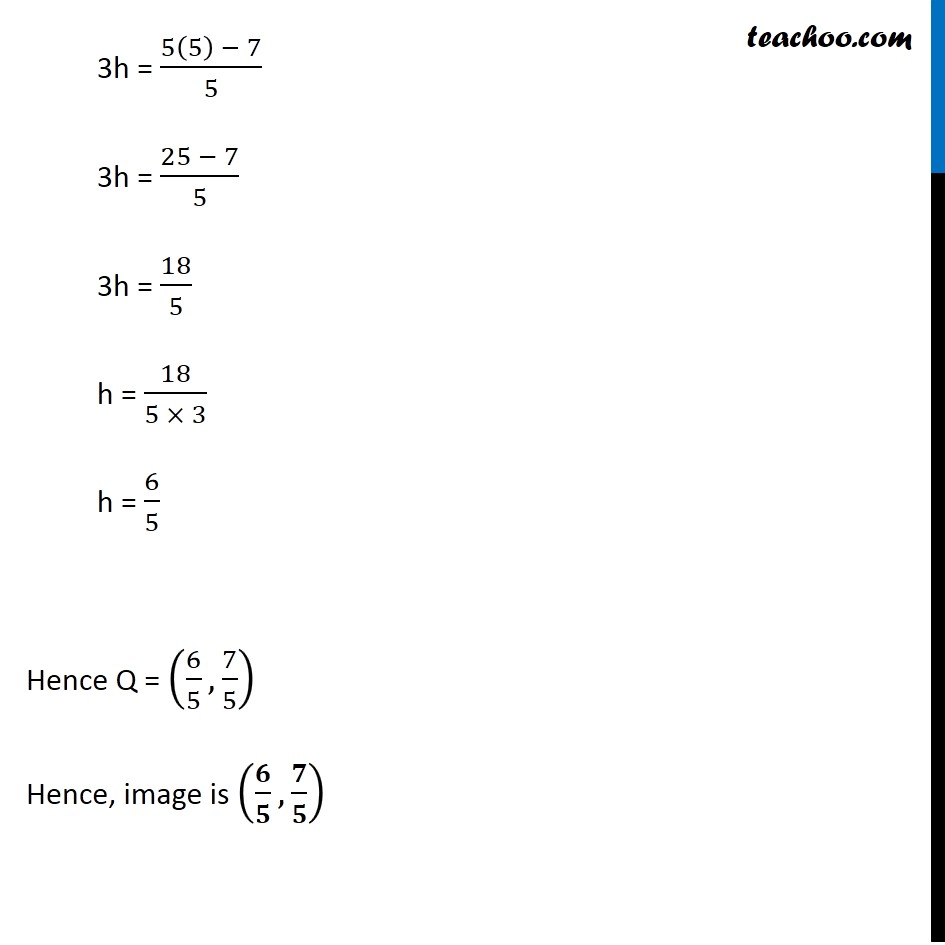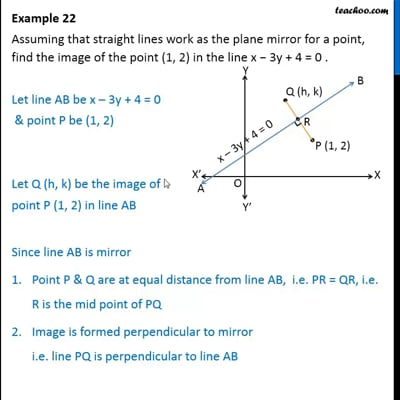This video is only available for Teachoo black users

Solve all your doubts with Teachoo Black (new monthly pack available now!)

### Transcript

Example 22 Assuming that straight lines work as the plane mirror for a point, find the image of the point (1, 2) in the line x − 3y + 4 = 0 . Let line AB be x – 3y + 4 = 0 & point P be (1, 2) Let Q (h, k) be the image of point P (1, 2) in line AB Since line AB is mirror Point P & Q are at equal distance from line AB, i.e. PR = QR, i.e. R is the mid point of PQ Image is formed perpendicular to mirror i.e. line PQ is perpendicular to line AB Since R is the mid point of PQ We know that mid point of a line joining (x1, y1) & (x2, y2) = ((𝑥_1+〖 𝑥〗_2)/2, (𝑦_1+ 𝑦_2)/2) Mid point of PQ joining (1, 2) & (h, k) is = ((1 + ℎ)/2 ", " (2 + 𝑘)/2) Coordinate of point R = ((1 + ℎ)/2 ", " (2 + 𝑘)/2) Since point R lies on the line AB It will satisfy the equation of line AB Putting x = (ℎ + 1)/2 & y = (𝑘 + 2)/2 in equation of AB x – 3y + 4 = 0 ((ℎ + 1)/2) – 3((𝑘 + 2)/2) + 4 = 0 (ℎ + 1 − 3(𝑘 + 2) + 4 × 2)/2 = 0 h + 1 – 3k – 6 + 8 = 0 h – 3k + 1 – 6 + 8 = 0 h – 3k + 3 = 0 h – 3k = –3 Also, PQ is perpendicular to AB We know that If two lines are perpendicular then product of their slope is equal to – 1 ∴ Slope of AB × Slope of PQ = –1 Slope of PQ = (−1)/(𝑆𝑙𝑜𝑝𝑒 𝑜𝑓 𝐴𝐵) Finding slope of AB Equation of line AB is x – 3y + 4 = 0 x + 4 = 3y 3y = x + 4 y = (𝑥 + 4)/3 y = (1/3)x + 4/3 The above equation is of the form y = mx + c where m = Slope of line So, Slope of line AB = 1/3 Now, Slope of line PQ = (−1)/(𝑆𝑙𝑜𝑝𝑒 𝑜𝑓 𝐴𝐵) = (−1)/(1/3) = –3 Now, Line PQ is formed by joining points P(1, 2) & Q(h, k) Slope of PQ = (𝑦_2 − 𝑦_1)/(𝑥_2− 𝑥_1 ) –3 = (𝑘 − 2)/(ℎ − 1) –3(h – 1) = k – 2 –3h + 3 = k – 2 –3h – k = –2 – 3 –3h – k = –5 –(3h + k) = –5 3h + k = 5 Now, our equations are h – 3k = –3 & 3h + k = 5 From (1) h – 3k = –3 h = 3k – 3 Putting value of h in (2) 3h + k = 5 3(3k – 3) + k = 5 9k – 9 + k = 5 9k + k = 5 + 9 10k = 14 k = 14/10 k = 7/5 Putting k = 7/5 in (1) 3h + k = 5 3h + 7/5 = 5 3h = 5 – 7/5 3h = (5(5) − 7)/5 3h = (25 − 7)/5 3h = 18/5 h = 18/(5 × 3) h = 6/5 Hence Q = (6/5, 7/5) Hence, image is (𝟔/𝟓, 𝟕/𝟓)